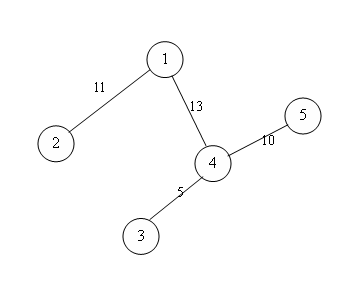﻿ 【POJ 3585 Accumulation Degree】 stdKonjac的小站

## 【POJ 3585 Accumulation Degree】

### 题目链接

http://poj.org/problem?id=3585

### Description

Trees are an important component of the natural landscape because of their prevention of erosion and the provision of a specific ather-sheltered ecosystem in and under their foliage. Trees have also been found to play an important role in producing oxygen and reducing carbon dioxide in the atmosphere, as well as moderating ground temperatures. They are also significant elements in landscaping and agriculture, both for their aesthetic appeal and their orchard crops (such as apples). Wood from trees is a common building material.

Trees also play an intimate role in many of the world’s mythologies. Many scholars are interested in finding peculiar properties about trees, such as the center of a tree, tree counting, tree coloring. A(x) is one of such properties.

A(x) (accumulation degree of node x) is defined as follows:

1. Each edge of the tree has an positive capacity.
2. The nodes with degree of one in the tree are named terminals.
3. The flow of each edge can’t exceed its capacity.
4. A(x) is the maximal flow that node x can flow to other terminal nodes.

Since it may be hard to understand the definition, an example is showed below:A(1)=11+5+8=24
Details:

1->2 11

1->4->3 5
1->4->5 8(since 1->4 has capacity of 13)
A(2)=5+6=11
Details:

2->1->4->3 5
2->1->4->5 6
A(3)=5
Details: 3->4->5 5
A(4)=11+5+10=26
Details:

4->1->2 11
4->3 5
4->5 10
A(5)=10
Details:

5->4->1->2 10
The accumulation degree of a tree is the maximal accumulation degree among its nodes. Here your task is to find the accumulation degree of the given trees.

### Input

The first line of the input is an integer T which indicates the number of test cases. The first line of each test case is a positive integer n. Each of the following n – 1 lines contains three integers xyz separated by spaces, representing there is an edge between node x and node y, and the capacity of the edge is z. Nodes are numbered from 1 to n.
All the elements are nonnegative integers no more than 200000. You may assume that the test data are all tree metrics.

### Output

For each test case, output the result on a single line.

1
5
1 2 11
1 4 13
3 4 5
4 5 10

### Sample Output

26

$$D[i]=\sum\limits_{j=1}^{sonNum[i]}min(D[j],e[i][j].capacity),j为非叶子节点;e[i][j].capacity,j为叶子节点$$。### 留下你的评论

*评论支持代码高亮<pre class="prettyprint linenums">代码</pre>